# Planting Example¶

Below I create a code example where I plant a region containing 5% of the points and make this region anomalous. Inside of the region 80% of the points are from the red set while outside of the region 50% of the points are red. We would like to recover this planted region by using the max_halfplane scanning algorithm.

In :

import pyscan
import matplotlib.pyplot as plt
import numpy as np
import random

def get_coord(i, lst):
return [pt[i] for pt in lst]

# l = (a, b, c) where ax + by + c = 0 => (-ax -c) / b = y
def f(mr, x):
l = mr.get_coords()
return -(x * l + l) / l

def plot_plane(red, blue, max_region, eps):

n = np.rint(1 / eps)
s = np.rint(1 / (2 * eps * eps))
# create a region containing 5% of the points. Inside of this region points are more likely to be red.
#print(get_coord(1, red))
_, ax = plt.subplots(figsize=(16, 12))
ax.scatter(get_coord(0, red), get_coord(1, red), marker=".", c="r")
ax.scatter(get_coord(0, blue), get_coord(1, blue), marker=".", c="b")

net = pyscan.my_sample(red, n) + pyscan.my_sample(blue, n)
red_s = pyscan.my_sample(red, s)
blue_s = pyscan.my_sample(blue, s)
approx_region, _ = pyscan.max_halfplane(net, red_s, blue_s, pyscan.KULLDORF)

ax.scatter(get_coord(0, net), get_coord(1, net), marker="x", c="k")
ax.plot([0, 1], [f(max_region, 0), f(max_region, 1)], c="k", linewidth=4.0)
ax.plot([0, 1], [f(approx_region, 0), f(approx_region, 1)], c="g", linewidth=4.0)
plt.xlim([0, 1])
plt.ylim([0, 1])
plt.axis('off')
plt.show()



We can plant a region that roughly cuts the point set in half and set two different halfs to be radically different. One side will have 90% percent red and the the other side 90% blue. The cutting region is in black.

In :

pts = [pyscan.WPoint(1.0, random.random(), random.random(), 1.0) for i in range(400)]
red, blue, max_region = pyscan.plant_halfplane(pts, .5, .1, .9)
_, ax = plt.subplots(figsize=(16, 12))

ax.scatter(get_coord(0, red), get_coord(1, red), marker=".", c="r")
ax.scatter(get_coord(0, blue), get_coord(1, blue), marker=".", c="b")
ax.plot([0, 1], [f(max_region, 0), f(max_region, 1)], c="k", linewidth=4.0)
ax.set_xlim([0, 1])
ax.set_ylim([0, 1])
plt.axis('off')
plt.show()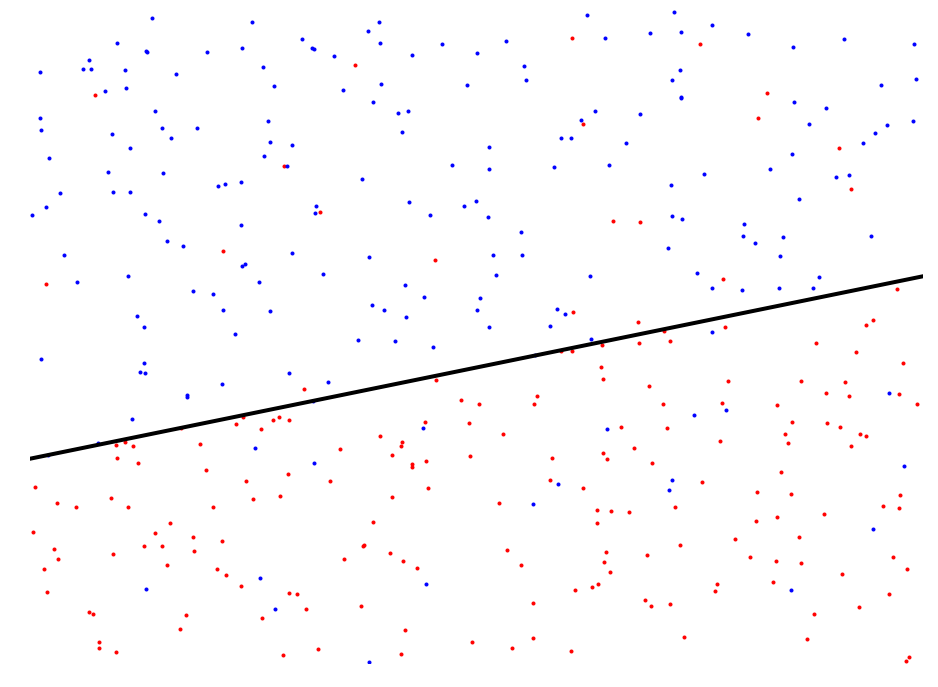As we use a larger sample size we get better and better results. The algorithm will subsample the data based on an error parameter called $$\varepsilon$$. There will be two samples uses in the algorithm one of size $$O(1/\varepsilon)$$ and another of size $$O(1/\varepsilon^2)$$. If we do not set $$\varepsilon$$ to be small enough we will not recover the region. Below I steadily decrease $$\varepsilon$$ and therefore increase the sample sizes. The small x markers define the regions that we scan. At first we will not recover the anomalous region.

In :

plot_plane(red, blue, max_region, .2)
plot_plane(red, blue, max_region, .1)In the above example we found the halfplane rather easily, but we can devise much more complicated situations where the region is much harder to recover.

In :

# generate some random points
pts = [pyscan.WPoint(1.0, random.random(), random.random(), 1.0) for i in range(10000)]
red, blue, max_region = pyscan.plant_halfplane(pts, .05, .5, .8)
_, ax = plt.subplots(figsize=(18, 12))

ax.scatter(get_coord(0, red), get_coord(1, red), marker=".", c="r")
ax.scatter(get_coord(0, blue), get_coord(1, blue), marker=".", c="b")
ax.plot([0, 1], [f(max_region, 0), f(max_region, 1)], c="k", linewidth=4.0)
ax.set_xlim([0, 1])
ax.set_ylim([0, 1])
plt.axis('off')
plt.show()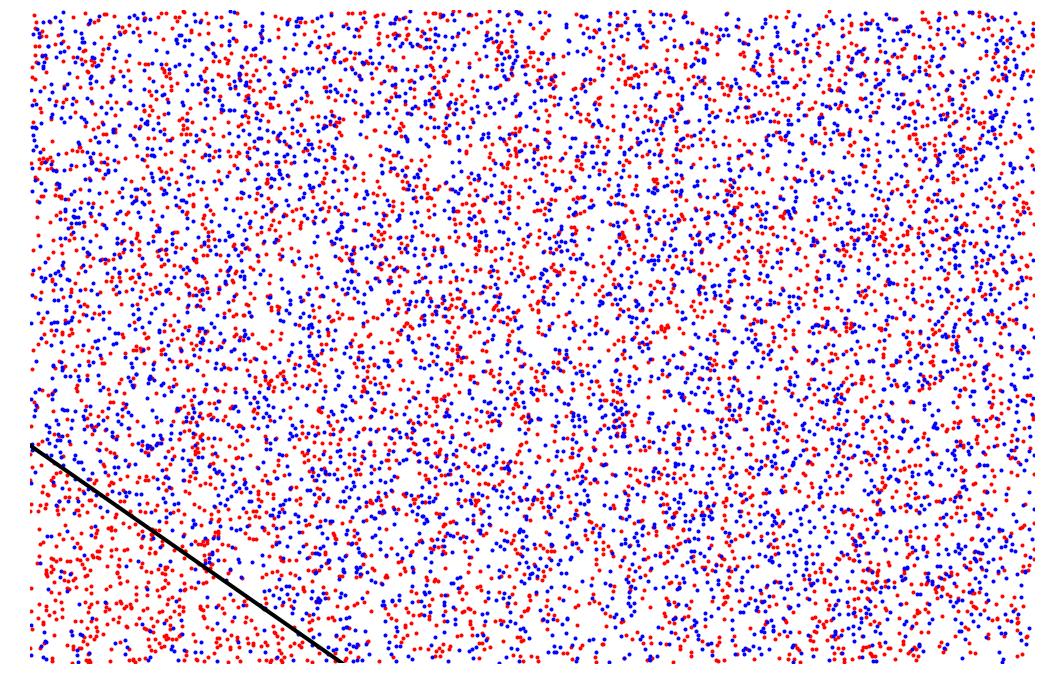Now we are scanning a point set with 10000 points and the region is much smaller and less anomalous.

In :

plot_plane(red, blue, max_region, .2)
plot_plane(red, blue, max_region, .1)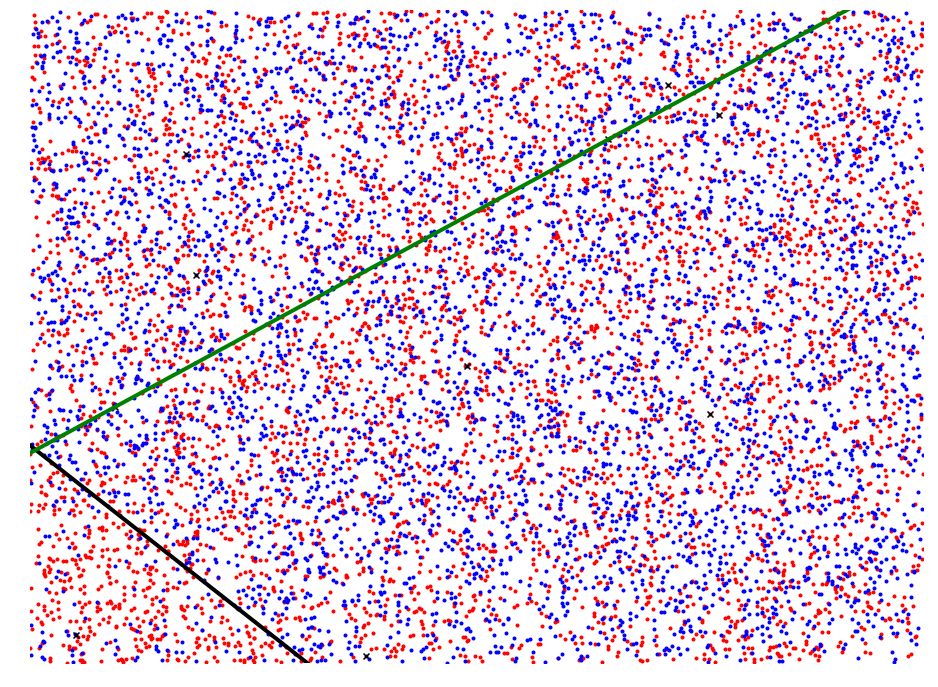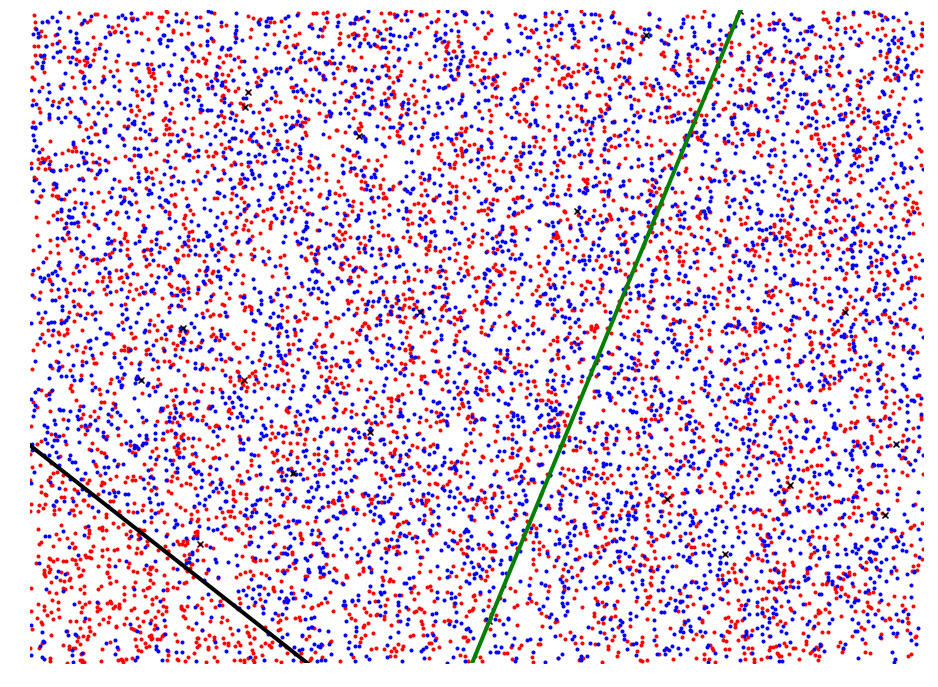In :

plot_plane(red, blue, max_region, .05)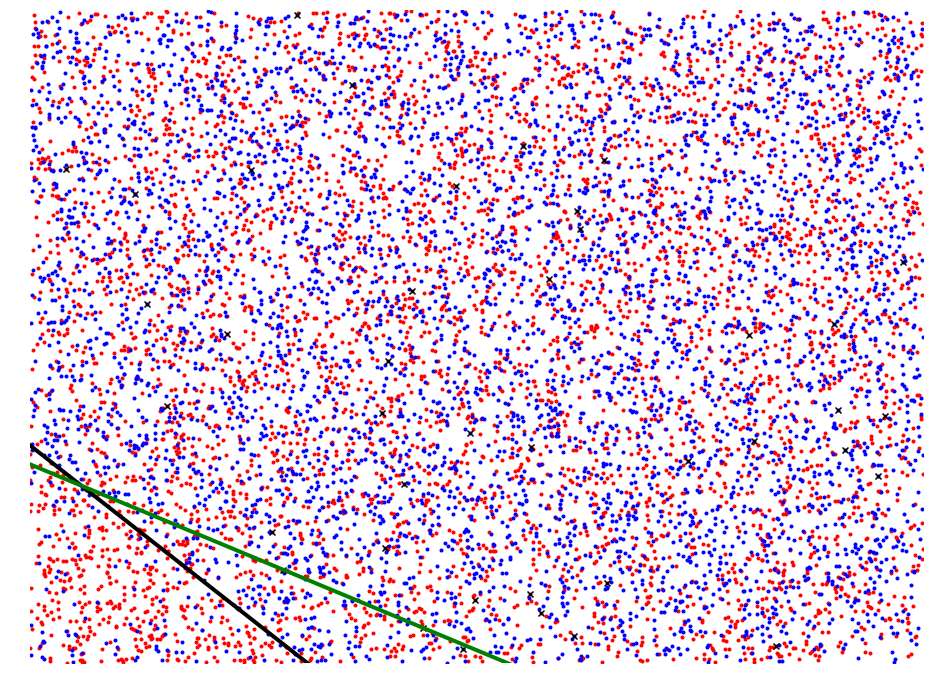Around .025 we get start getting very close to recovering the region and at .01 we almost perfectly recover it.

In :

plot_plane(red, blue, max_region, .025)
plot_plane(red, blue, max_region, .01)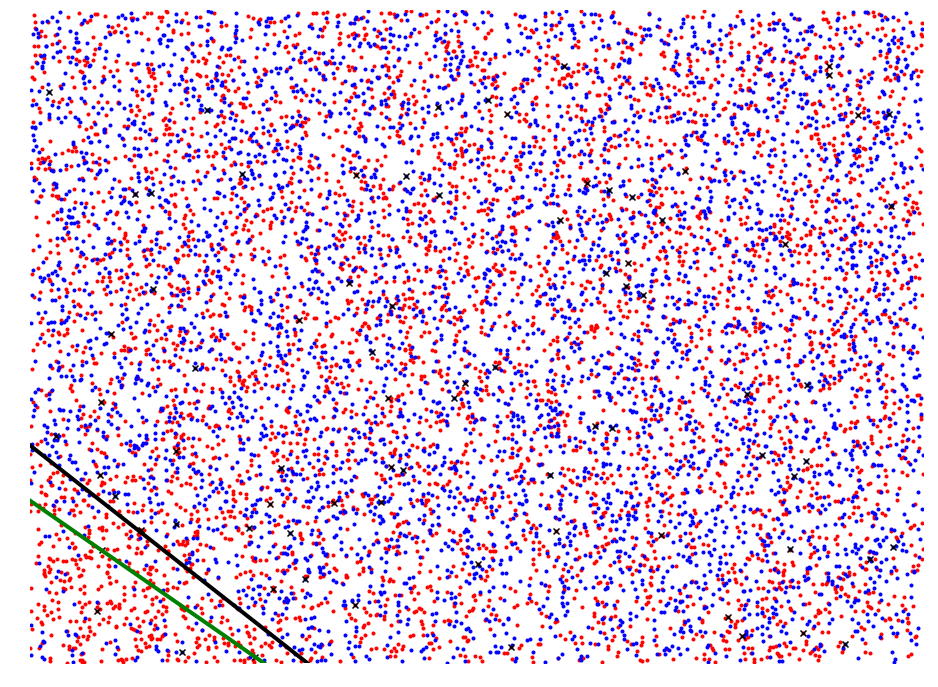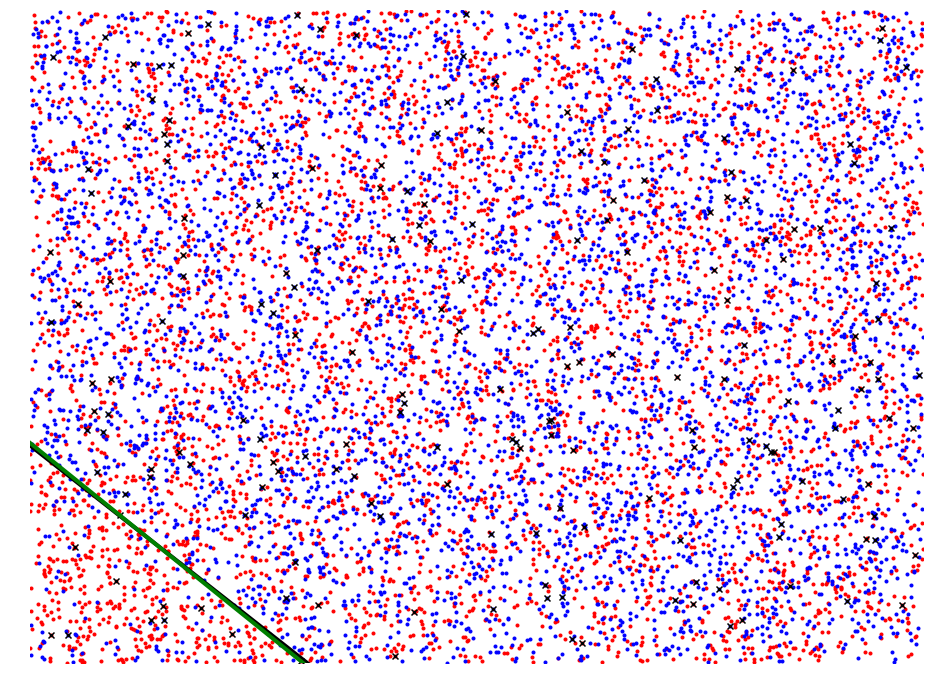We can plant a different where 60% of the points are from the anomalous red set and leave the baseline set at 50/50. This makes the region much harder to recover.

In :

red, blue, max_region = pyscan.plant_halfplane(pts, .05, .5, .6)

_, ax = plt.subplots(figsize=(16, 12))
plt.scatter(get_coord(0, red), get_coord(1, red), marker=".", c="r")
plt.scatter(get_coord(0, blue), get_coord(1, blue), marker=".", c="b")
plt.plot([0, 1], [f(max_region, 0), f(max_region, 1)], c="k", linewidth=4.0)
plt.xlim([0, 1])
plt.ylim([0, 1])
plt.axis("off")
plt.show()We now fail to recover the region with the earlier parameters that were able to recover the region.

In :

plot_plane(red, blue, max_region, .025)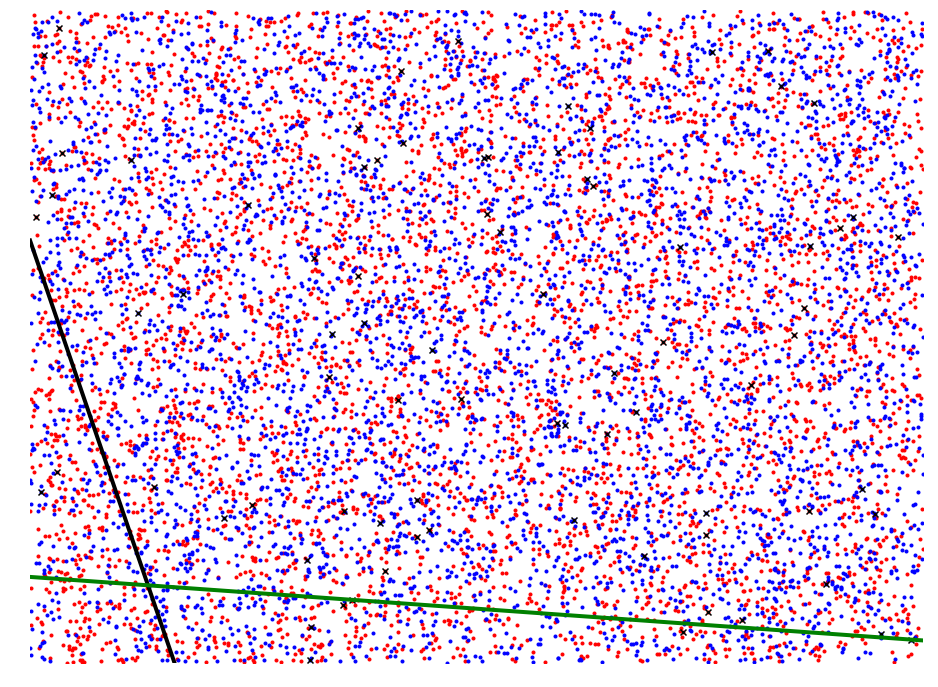Increasing the error paramter even more allows us to again recover the region.

In :

plot_plane(red, blue, max_region, .005)